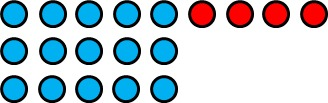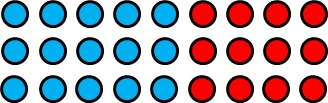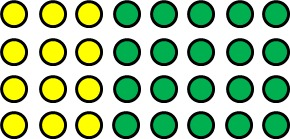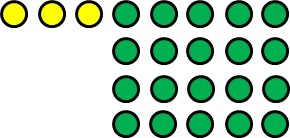# Why Do We Need an Order of Operations?

Alignments to Content Standards: 5.OA.A

1. State the meaning of each of the following expressions and draw a picture that represents it. $$(3\times5)+4$$ $$3\times(5+4)$$
2. State the meaning of this expression: $$3\times5+4$$ How do you know?
3. State the meaning of each of the following expressions and draw a picture that represents it. $$(3+5)\times4$$ $$3+(5\times4)$$
4. State the meaning of this expression: $$3+5\times4$$ How do you know?

## IM Commentary

The purpose of this task is to help students think about the reason for the mathematical convention known as the "order of operations." The task could be used in two ways: to present an apparently unresolvable mathematical situation that can lead to a classroom discussion about the need for an order of operations, or to help students who know already know the order of operations to pause and think more deeply about why they are necessary. For students to get the full benefit out of this task, they will need to hear a bit more from the teacher about the idea of mathematical conventions and why we need to have them when a mathematical statement would otherwise be ambiguous.

Addition, subtraction, multiplication, and division are all operations that take two numbers (named the addends, the minuend and subtrahend, the factors, or the divisor and the dividend, respectively) and produce a single number (called the sum, the difference, the product, or the quotient, respectively)--this is why they are called binary operations. In principle, grouping symbols are needed whenever there are more than 2 numbers that will be combined using one or more of these binary operations. This is because it is impossible to know a priori whether, for example,

$$8 + 3 \times 2$$

means we should add the 8 and the 3 first, which seems reasonable since in many languages, including English, we read from left to right, or multiply the 3 and the 2 first. In mathematics, we resolve the meaning of numeric expressions that include more than two numbers but do not contain grouping symbols in one of two ways:

• We convince ourselves that, in some instances, the result is the same regardless of which pair of numbers we choose to combine first.

• We choose conventions for the order in which we evaluate a numeric expression because we will get different results if we simplify the expressions in different ways.

$$a + (b + c) = (a + b) + c$$

and the associative property of multiplication:

$$a \times (b \times c) = (a \times b) \times c$$

are examples of the first of these. The so-called "order of operations" are how we have settled the second of these. To go back to our original example, we know that

$$8 + 3 \times 2 = 8 + (3 \times 2)$$

because we have all agreed that if we have an expression with at least three numbers that are combined using addition and multiplication, we will multiply before we add. There are actually some good mathematical reasons we have structured the order of operations as we have; to read more about it, see "Order of operations" and other oddities in school mathematics by H. Wu.

## Solution

1. The expression $(3\times5)+4$ means we should multiply 3 and 5 and then add 4 to the result. Here is a picture:The expression $3\times(5+4)$ means we should multiply 3 by the sum of 5 and 4. Here is a picture:2. The expression $3\times5+4$ means the same thing as the expression $(3\times5)+4$. If we haven't already learned about the order of operations, then it is possible for $$3\times5+4$$ to mean either $$(3\times5)+4$$ or $$3\times(5+4)$$ The only way to know what $3\times5+4$ means is to understand that we have all agreed that when we don't have grouping symbols, we should multiply before we add.
3. The expression $(3+5)\times4$ means we should add 3 and 5 and then multiply the result by 4. Here is a picture:The expression $3+(5\times4)$ means we should add 3 to the product of 5 and 4. Here is a picture:4. The expression $3+5\times4$ means the same thing as the expression $3+(5\times4)$. If we haven't already learned about the order of operations, then it is possible for $$3+5\times4$$ to mean either $$(3+5)\times4$$ or $$3+(5\times4)$$ The only way to know what $3+5\times4$ means is to understand that we have all agreed that when we don't have grouping symbols, we should multiply before we add.